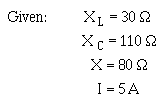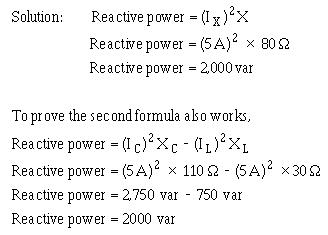voltage and current associated with the circuit reactance. ">Custom SearchCalculating Reactive Power in AC Circuits The reactive power is the power returned to the source by the reactive components of the circuit. This type of power is measured in Volt-Amperes-Reactive, abbreviated var. Reactive power is calculated by using the voltage and current associated with the circuit reactance. Since the voltage of the reactance is equal to the reactance multiplied by the reactive current, reactive power can be calculated by the formula:Another way to calculate reactive power is to calculate the inductive power and capacitive power and subtract the smaller from the larger.Either one of these formulas will work. The formula you use depends upon the values you are given in a circuit. For example, find the reactive power of the circuit shown in figure 4-10.Since this is a series circuit, current (I) is the same in all parts of the circuit.Q.22 What is the reactive power in an ac circuit? Q.23 What is the unit of measurement for reactive power? Q.24 What is the formula for computing reactive power?Integrated Publishing, Inc. - A (SDVOSB) Service Disabled Veteran Owned Small Business